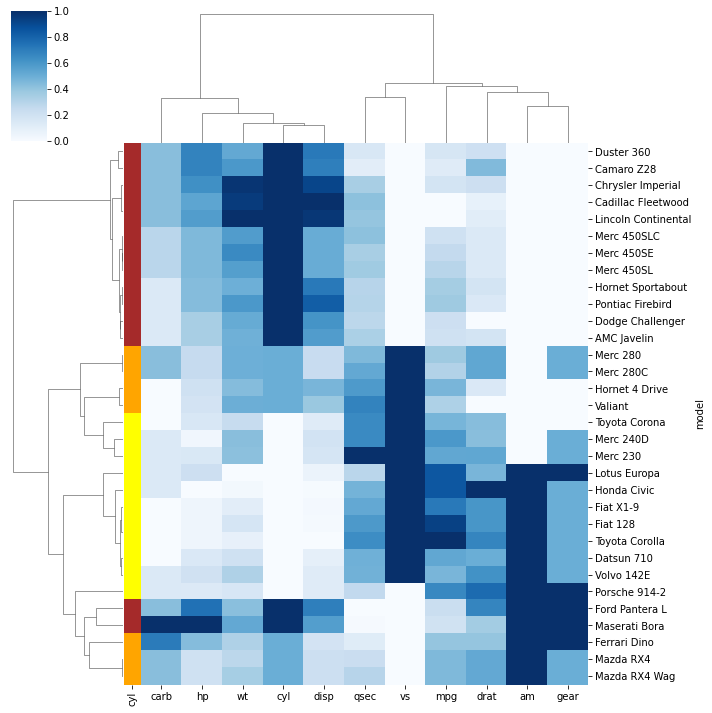In the example, mtcars dataset that shows the features of cars through numerical variables is used. While clustering cars, a color sheme is added to the left part of the plot. The 3 colours represent the 3 possible values of the ‘cyl’ column. By using this feature, you can evaluate whether samples within a group are clustered together.

``````# Libraries
import seaborn as sns
import pandas as pd
from matplotlib import pyplot as plt

# Data set
url = 'https://raw.githubusercontent.com/holtzy/The-Python-Graph-Gallery/master/static/data/mtcars.csv'
df = df.set_index('model')

# Prepare a vector of color mapped to the 'cyl' column
my_palette = dict(zip(df.cyl.unique(), ["orange","yellow","brown"]))
row_colors = df.cyl.map(my_palette)

# plot
sns.clustermap(df, metric="correlation", method="single", cmap="Blues", standard_scale=1, row_colors=row_colors)
plt.show()``````## Contact & Edit

👋 This document is a work by Yan Holtz. You can contribute on github, send me a feedback on twitter or subscribe to the newsletter to know when new examples are published! 🔥